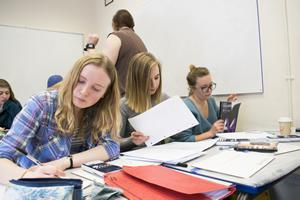# Mathematics

#### OCR (MEI)Doing well in A Level Mathematics requires you to make connections, understand why what you do works and adapt your thinking to a wide range of problems.  This is much more interesting than just learning more techniques.

## Course Content

The course assumes students arrive with good algebra skills and a good understanding of graphs.  This is essentially the level 7-9 work at GCSE.  We will begin by extending some methods from GCSE.

Problem solving, modelling and mathematical reasoning are the key themes of A level Mathematics, which will require you to think flexibly, be prepared to experiment and evaluate results.

Year 1:
Pure

• Proof
• Coordinate geometry, including circles
• Trigonometry
• Differentiation and integration
• Vectors
• Exponentials and logarithms

Statistics

• Data representation and presentation
• Probability
• Binomial distribution
• Hypothesis testing

Mechanics

• Kinematics (describing and quantifying motion)
• Forces and Newton’s laws of motion
• Variable acceleration

Two thirds of the A level is pure mathematics; statistics and mechanics are one sixth each.

Year 2:

Year 2 has a similar structure and the content naturally builds on that of Year 1.  The very important Normal distribution is covered in statistics.  Mechanics will focus more on modelling situations in 2-dimensions.## Where Could It Take Me?

A level Mathematics is essential for those studying courses with a high level of mathematical content, e.g. Mathematics, Statistics, Engineering, Computer Science and Economics.

A good Mathematics A level grade is also required by some universities for Computer Science and Economics.

Students wishing to apply for Maths, Engineering, Computer Science or Physics at the most selective universities would also find an A level in Further Maths either essential or advantageous.

## Entry Requirements

5 GCSEs at grade 4 or above, including English and a grade 7 or above in Mathematics. Good algebraic skills are essential and a willingness to think.

## Methods of Teaching

Lessons will be varied, mixing investigations, class discussions, checking progress with mini-white boards, working collaboratively and completing the essential practice that will perfect your understanding.  It is vital that you use your study periods to complete problems and review ideas.  The graphical calculator is a great tool for deepening our understanding, checking working and saving time.  You will have opportunities to develop your calculator skills in lessons and your teacher will show you how to make effective use of the department website and other online resources.  The subject content will be assessed regularly by means of tests and assignments.

There is a well-resourced workshop, for additional support, every lunchtime. Teachers and upper sixth students will be present at each session to offer you help.

## Methods & Patterns of Assessment

There will be three 2 hour external exams in May/June at the end of year two.  There is no coursework.

• Paper 1 is a mix of pure maths and mechanics.  The questions will be arranged in increasing level of difficulty, rather than separated into pure and mechanics.
• Paper 2 is a mix of pure maths and statistics, with questions arranged in increasing level of difficulty rather than by topic.  Some of the statistics questions will refer to a large data set, which you will need to have spent some time analysing during the course.
• Paper 3 will be on pure mathematics only and will include a comprehension section, where you will answer questions based on a previously unseen mathematical article.

50% of the marks are for problem solving, modelling and mathematical reasoning.

A level Mathematics requires calculator functions beyond that of the standard GCSE scientific calculators.  We insist our students have a specific graphical calculator, the Casio fx-CG50. It is best to wait until you are at college where you can buy the fx-CG50 at a reduced price.

## Financial Implications

You will need to purchase your own textbooks, stationery and the required graphical calculator.  In some cases, where there is difficulty meeting these costs, the college student support fund may help.